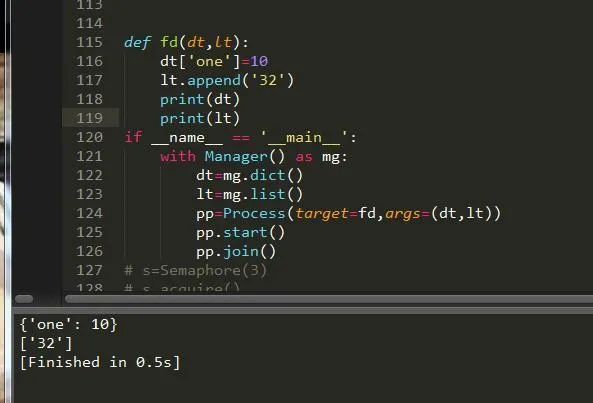# Python基础之进程详解

## 一、前言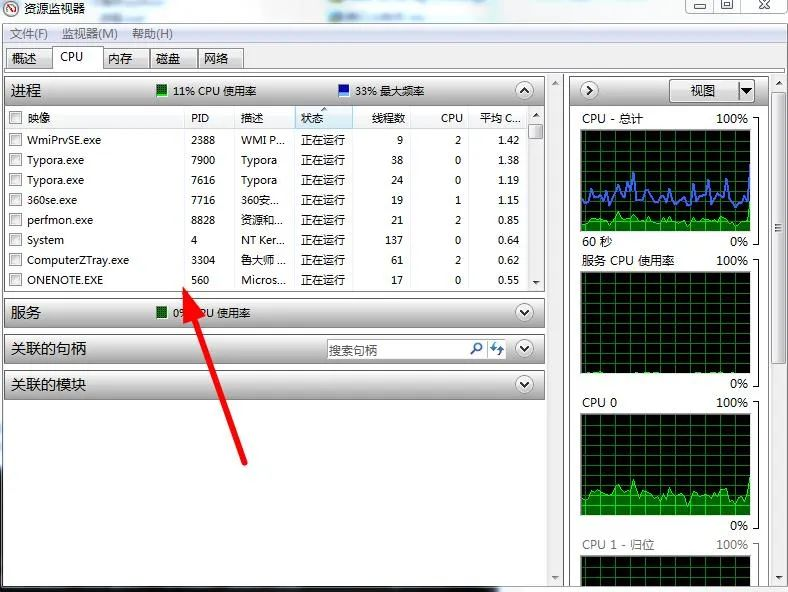## 二、基本用法

 12 `import` `multiprocess as m``m.Process(target，args)`

 1234567 `from` `multiprocessing ``import` `Process``Process(group, target, args, kwargs, name)``group:用户组``target:调用函数``args:参数元祖``kwargs:参数字典``name:子进程名称`

 123456 `# 返回当前进程存活的子进程的列表。调用该方法有“等待”已经结束的进程的副作用。``multiprocessing.active_children()`` ` ` ` `# 返回系统的CPU数量。``multiprocessing.cpu_count()`

## 三、创建单个进程

 1234567891011121314151617181920212223242526272829303132333435363738394041424344454647484950 `#启动进程，调用进程中的run()方法。``start()`` ` ` ` `#进程活动的方法``run()`` ` ` ` `#强制终止进程，不会进行任何清理操作。如果终止前创建了子进程，那么该子进程在其强制结束后变为僵尸进程；如果该进程还保存了一个锁，那么也将不会被释放，进而导致死锁。``terminate()`` ` ` ` `#判断某进程是否存活，存活返回True，否则False。``is_alive()`` ` ` ` `主线程等待子线程终止。timeout为可选择超时时间；需要强调的是：p.join只能join住start开启的进程，而不能join住run开启的进程。``join([timeout])`` ` ` ` `#设置进程为后台守护进程；当该进程的父进程终止时，该进程也随之终止，并且该进程不能创建子进程，设置该属性必须在start()之前``daemon`` ` ` ` `#进程名称。``name`` ` ` ` `#进程pid，在start后才能产生``pid`` ` ` ` `#子进程的退出代码。如果进程尚未终止，这将是 None，负值-N表示子进程被信号N终止。``exitcode`` ` ` ` `#进程身份验证，默认是os.urandom()随机生成的字符串。校验网进程连接是否正确``authkey`` ` ` ` `#系统对象的数字句柄，当进程结束时将变为 "ready" 。``sentinel`` ` ` ` `#杀进程``kill()`` ` ` ` `#关闭进程``close()`

 1 `if` `__name__ ``=``=` `'__main__'``:`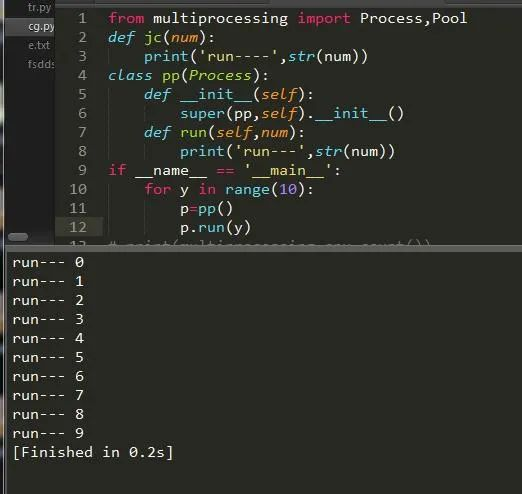## 四、创建多个进程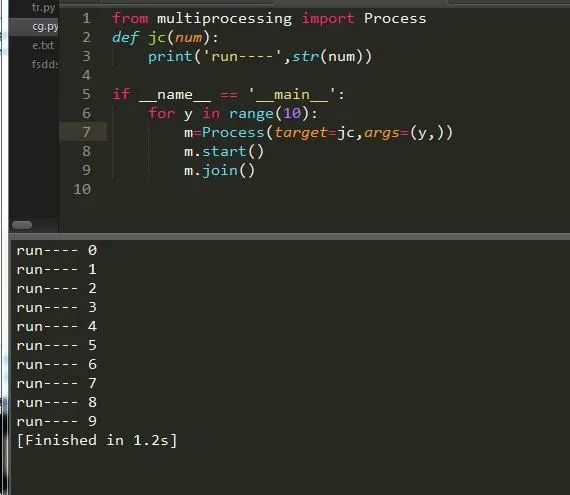## 五、进程池

 12 `from` `multiprocessing ``import` `Pool``import` `multiprocessing as m`

 1 `num``=``m.cpu_count()``#CPU内核数`

 1 `pool``=``multiprocessing.Pool(num)`

 12345678910111213141516171819202122232425262728293031 `apply``(func，args，kwargs)                 同步执行（串行） 阻塞`` ` ` ` `apply_async(func，args，kwargs)           异步执行（并行） 非阻塞`` ` ` ` `terminate()            强制终止进程，不在处理未完成的任务。`` ` ` ` `join()     主进程阻塞，等待子进程的退出。必须在close或terminate()之后使用`` ` ` ` `close()            等待所有进程结束后，才关闭进程池`` ` ` ` `map``(func，iterable，chunksize``=``int``) ``map``函数的并行版本，保持阻塞直到获得结果`` ` ` ` `#返回一个可用于获取结果的对象，回调函数应该立即执行完成，否则会阻塞负责处理结果的线程``map_async(func，iterable，chunksize，callback，error_callback)`` ` ` ` `imap(func，iterable，chunksize) ``map``的延迟执行版本`` ` ` ` `#和imap() 相同，只不过通过迭代器返回的结果是任意的``imap_unordered(func，iterable，chunksize)`` ` ` ` `#和 map() 类似，不过 iterable 中的每一项会被解包再作为函数参数。``starmap(func，iterable，chunksize)`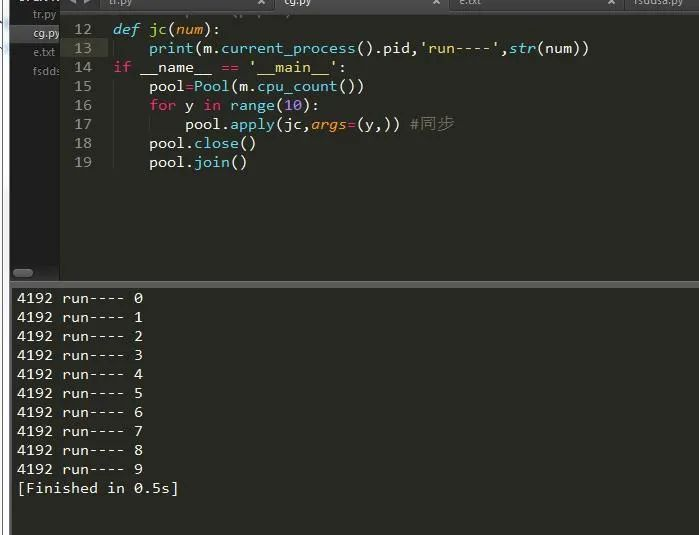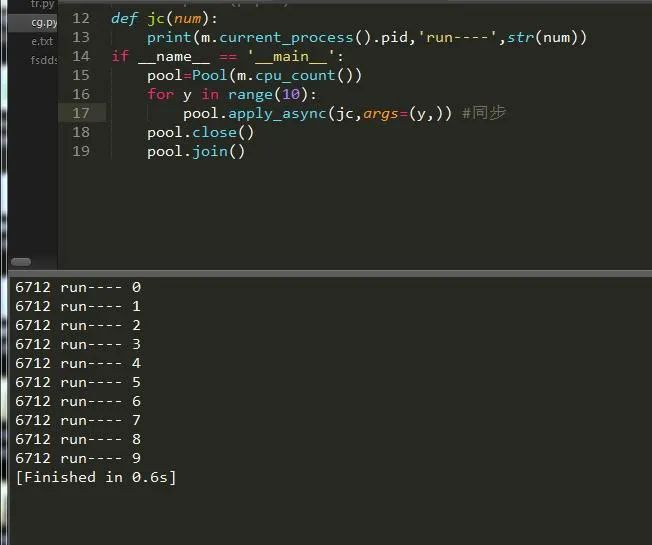## 六、锁

 1 `from` `multiprocessing ``import` `Lock`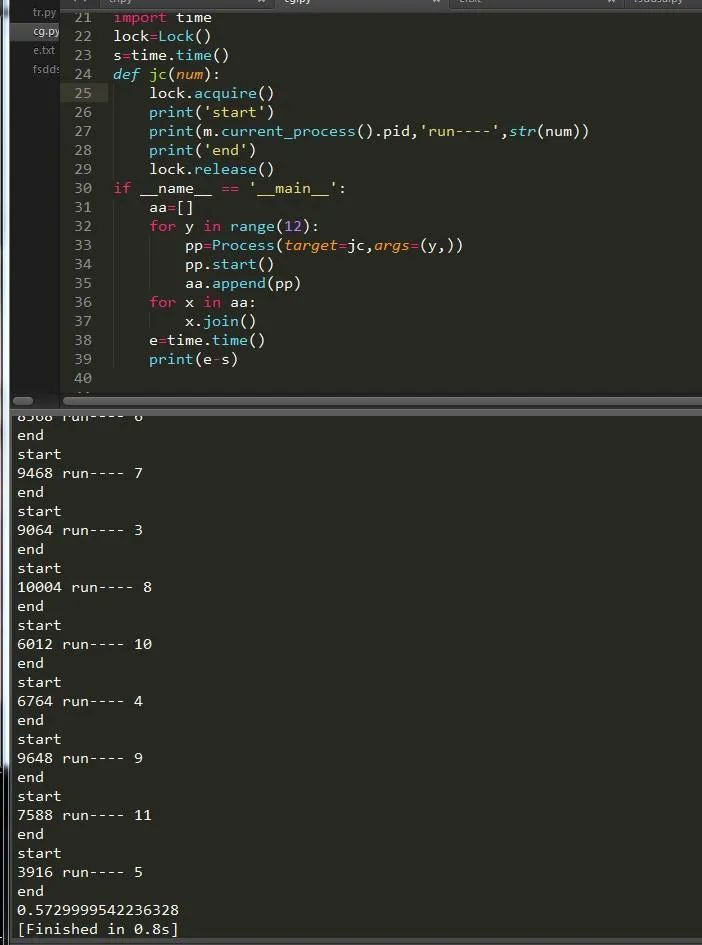12345678910111213141516171819202122 `import` `time``lock1``=``RLock()``lock2``=``RLock()``s``=``time.time()``def` `jc(num):``    ``lock1.acquire()``    ``lock2.acquire()``    ``print``(``'start'``)``    ``print``(m.current_process().pid，``'run----'``，``str``(num))``    ``lock1.release()``    ``lock2.release()``    ``print``(``'end'``)``if` `__name__ ``=``=` `'__main__'``:``    ``aa``=``[]``    ``for` `y ``in` `range``(``12``):``        ``pp``=``Process(target``=``jc，args``=``(y，))``        ``pp.start()``        ``aa.append(pp)``    ``for` `x ``in` `aa:``        ``x.join()``    ``e``=``time.time()``    ``print``(e``-``s)`

## 七、进程间通信

Event

 123456789101112131415161718192021222324 `import` `time``e``=``Event()``def` `main(num):``    ``while` `True``:``        ``if` `num<``5``:``            ``e.clear()   ``#清空信号标志``            ``print``(``'清空'``)``        ``if` `num>``=``5``:``            ``e.wait(timeout``=``1``) ``#等待信号标志为真``            ``e.``set``()``            ``print``(``'启动'``)``            ` `        ``if` `num``=``=``10``:``            ``e.wait(timeout``=``3``)``            ``e.clear()``            ``print``(``'退出'``)``            ``break``        ``num``+``=``1``        ``time.sleep(``2``)``if` `__name__ ``=``=` `'__main__'``:``    ``for` `y ``in` `range``(``10``):``        ``pp``=``Process(target``=``main，args``=``(y，))``        ``pp.start()``        ``pp.join()`

 1 `p1，p2``=``m.Pipe(duplex``=``bool``) ``#设置是否全双工，返回两个连接对象`
 123456789 `p1.send()  ``#发送``p2.recv()  ``#接收``p1.close()  ``#关闭连接``p1.fileno() ``#返回连接使用的整数文件描述符``p1.poll([timeout]) ``#如果连接上的数据可用，返回True，timeout指定等待的最长时限。``p2.recv_bytes([maxlength]) ``#接收最大字节数``p1.send_bytes([maxlength]) ``#发送最大字节数``#接收一条完整的字节消息，并把它保存在buffer对象中，offset指定缓冲区中放置消息处的字节位移.``p2.recv_bytes_into(``buffer` `[， offset])`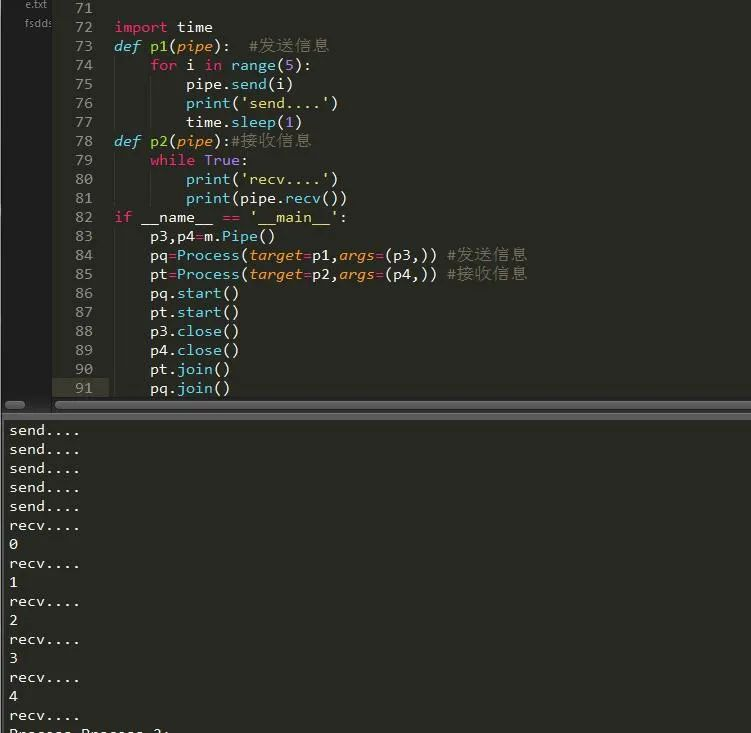12345678910111213141516 `def` `fd(a):``    ``for` `y ``in` `range``(``10``):``        ``a.put(y)  ``#插入数据``        ``print``(``'插入：'``，``str``(y))``def` `df(b):``    ``while` `True``:``        ``aa``=``b.get(``True``) ``#删除数据``        ``print``(``'释放：'``，``str``(aa))``if` `__name__ ``=``=` `'__main__'``:``    ``q``=``Queue()``    ``ff``=``Process(target``=``fd，args``=``(q，))``    ``dd``=``Process(target``=``df，args``=``(q，))``    ``ff.start() ``#开始运行``    ``dd.start()``    ``dd.terminate() ``#关闭``    ``ff.join()`

## 八、信号量

 12345678910111213141516 `s``=``Semaphore(``3``)``s.acquire()``print``(s.get_value())``s.release()``print``(s.get_value())``print``(s.get_value())``s.release()``print``(s.get_value())``s.release()`` ` ` ` `output：``2``3``3``4`

## 九、数据共享

 1234 `数值型：m.Value()  ``数组性：m.Array()  ``字典型：m.``dict``()  ``列表型:m.``list``()`

 12 `Manager().``dict``()``Manager.``list``()`